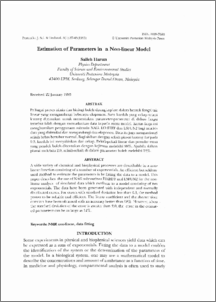# Estimation of parameters in a non-linear model

## Citation

Harun, Salleh (1995) Estimation of parameters in a non-linear model. Pertanika Journal of Science & Technology, 3 (1). pp. 37-43. ISSN 0128-7680

## Abstract

A wide variety of chemical and biophysical processes are describable in a nonlinear function consisting of a number of exponentials. An efficient but seldom used method to estimate the parameters is by fitting the data to a model. This paper describes the use of NAG subroutines E04HFF and LSFUN2 for the nonlinear analysis of simulated data which conform to a model consisting of two exponentials. The data have been generated with independent and normally distributed errors. For errors with standard deviation less than 0.3, the method proves to be reliable and efficient. The linear coefficient and the shorter time constant have been obtained with an accuracy better than 98%. However, when the standard deviation of the error is greater than 2.0, the error in the estimated parameters can be as large as 12%.Preview
PDF
Estimation_of_Parameters_in_aNon-linear_Model.pdfView Item